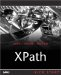# Boolean Functions

As you know, there are no explicit values named true and false that you can embed in XPath 2.0 expressions. Instead, if you need a true or false value, you use the Boolean constructor functions fn:true and fn:false . In addition, the fn:not function flips the logical value of its argument. Here are the Boolean functions in overview:

• fn:true returns the xs:boolean value true .

• fn:false returns the xs:boolean value false .

• fn:not flips the Boolean value of its argument, that is, if passed true it returns false ; if passed false it returns true.

We'll take a brief look at all three functions.

#### The fn:true Function

The fn:true() function has no other purpose than to return a xs:boolean value of true . Here's its signature:

` `
` fn:true() as xs:boolean `

Having a function that can return the xs:boolean value true can be useful at times because some XPath 2.0 functions require you to pass Boolean values of true or false .

#### The fn:false Function

This function simply returns the xs:boolean value false . Here's its signature:

` `
` fn:false() as xs:boolean `

We've already used this function in Chapter 10, when we used the fn: escape-uri function to escape a URI. In that example (Listing 10.12), we didn't want to escape every possible character, so we passed the fn:escape-uri function a value of false using fn:false :

` `
` <xsl:stylesheet version="2.0"     xmlns:xsl="http://www.w3.org/1999/XSL/Transform"     xmlns:xs="http://www.w3.org/2001/XMLSchema">     <xsl:template match="/">  <xsl:value-of select="escape-uri(   'http://www.XPathCorp/My Web Page.html', false())"/>  </xsl:template> </xsl:stylesheet> `

#### The fn:not Function

This function just flips the Boolean value of its argument. Here's how you use it:

` `
` fn:not(  \$srcval  as item*) as xs:boolean `

The sequence \$srcval is first converted to a xs:boolean value by passing it to the fn:boolean . We're going to see this function in Chapter 12it returns true unless you pass to it any of the following:

• The singleton xs:boolean value false .

• The singleton xs:string value "".

• A singleton numeric value that is numerically equal to zero.

• The singleton xs:double or xs:float value NaN ("Not a Number").

Otherwise, this function returns true . The result of xs:boolean is passed to the fn:not function, which flips true to false and false to true to yield the final value.

Here's an example; in this case, we'll check to make sure the temperature is not over 100 degrees Fahrenheit with this expression: if(not(\$temperature > 100)) then... . You can see this at work in ch11_01.xsl (Listing 11.1).

##### Listing 11.1 Using the fn:not Function ( ch11_01.xsl )
` <xsl:stylesheet version="2.0"     xmlns:xsl="http://www.w3.org/1999/XSL/Transform"     xmlns:xs="http://www.w3.org/2001/XMLSchema">     <xsl:variable name="temperature" select="65" />     <xsl:template match="/">  <xsl:value-of select="if(not(\$temperature > 100))   then 'Temperature is OK.'   else 'Too hot.'"/>  </xsl:template> </xsl:stylesheet> `

And here's the result, where we see that the handy fn:not function has indeed flipped the Boolean value of its argument:

` `
` <?xml version="1.0" encoding="UTF-8"?> Temperature is OK. `

This function is particularly useful when you're dealing with true / false values already stored in variables and you need to handle the reverse Boolean sense. For example, if a variable is named \$mortgageApproved and you need to create a letter for those cases where the mortgage was not approved, you can use the expression fn:not(\$mortgageApproved) .XPath Kick Start: Navigating XML with XPath 1.0 and 2.0
ISBN: 0672324113
EAN: 2147483647
Year: 2002
Pages: 131

Similar book on Amazon